## and Sounding Analysis

Dr. John W. (Jack) Glendening,  Meteorologist

27 February 2003

A note from the author
This webpage is intended for those pilots who want to better understand the atmosphere in which they fly, for those who want to interpret soundings (either observed or model generated) and their significance for a day's thermalling conditions, and for recipients of the Thermal Index Prediction (TIP) emails who wish to better understand the theory behind the TI method.  For these purposes, some understanding of the convective boundary layer is required.  This webpage is divided into two parts, with Part I giving basics of the convective boundary layer and Part II describing how plotted soundings can be analyzed.  Those who are primarily interested in how the BL works can read just Part I.  Part II contains some sections that are slanted towards the Thermal Index method used in my early TIP forecasts, but it is still generally applicable to all types of sounding analysis.  Note that for simplicity the example soundings use a basic "temperature vs. height" plot format, not the "skewT" plot format often used by meteorologists, but the principles involved are similar for all sounding plot formats.
While writing I find myself drowning in mental caveats, thinking "BUT ...".  Atmospheric processes can be complex: many variations can occur and seldom will simple descriptions cover all possibilities.  I have tried to include only the important caveats so as to not overwhelm the reader, so this present discussion is only an introduction.  A different approach to soundings and the Thermal Index is available at the Forecasting Thermals Made Easy website. A non-technical description of thermals and the convective boundary layer is given at the What do thermals look like? webpage.

## PART I:  CONVECTIVE BOUNDARY LAYER BASICS

### Basics of Atmospheric Stability (Lapse Rate)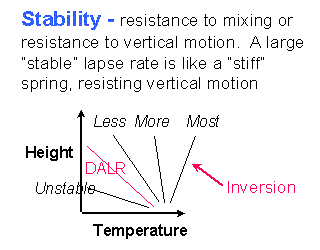The lapse rate  is the change of atmospheric temperature with height.  The lapse rate determines the atmosphere's resistance to vertical motion, or stability.  If the lapse rate equals the DALR [Dry Adiabatic Lapse Rate, which is -5.4°F per 1000 ft  with the negative sign indicating that temperature decreases with height] then there is no resistance to vertical motion.   If the lapse rate is larger, or "more stable"  the atmosphere resists vertical motion.  An atmosphere in which the temperature increases with height is called an inversion and it is very stable.  An atmosphere in which the lapse rate is smaller (more negative) than the DALR is "unstable" and tends to spontaneously mix.  The only region of the atmosphere which is generally unstable is the layer immediately above heated ground, in which thermals form.

### Basics of a Mixing Layer

If a layer of dry air is perfectly mixed, it will then have a lapse rate equal to the DALR.  The reason for this is that air is compressible and so pressure, which changes with height, also affects the resulting temperature [in contrast, if water - which is not compressible - is mixed its temperature is then constant with height, with a lapse rate equal to 0].  Mixing can be caused by a number of factors such as thermals, wind shear, and clouds.  In reality mixing is seldom perfect and the lapse rate is seldom exactly equal to the DALR over a deep layer.  Weak thermals, for example, do not produce as much mixing as do stronger thermals.  Nevertheless, the lapse rate in a mixed layer is often close to the DALR.  A temperature profile which exactly equals the DALR is called an adiabat.

### Virtual Temperature

This section is an aside and can be skipped.  It is provided to answer questions raised by the use of the term "virtual temperature" since the TIP sounding plot is, strictly speaking, actually in virtual temperature).  The discussion of the preceding sections is strictly true only for perfectly dry air, i.e. air without any humidity.  The density of water vapor [a gas, not a liquid - don't confuse this with liquid water and cloud formation!] is less than the density of dry air so adding humidity to air decreases its density.  Density is the critical factor affecting buoyancy and mixing, so somehow the change of density due to humidity must be accommodated.  This is done by defining virtual temperature to be the the temperature which dry air would have if its density were equal to that humid air.  The statements in the above paragraph are then true for the case of humid air when virtual temperature is used instead of regular temperature.

The TIP employs virtual temperature for its calculations and the TIP plots are also of virtual temperature.  However, the difference between virtual temperature and regular temperature is relatively small, usually less than 4°F.  (The virtual temperature will always be larger than the regular temperature because adding humidity always makes the air less dense.)  Also, the difference between the lapse rates using regular temperature and virtual temperature is very small.  So typically the difference between virtual temperature and regular temperature can be glossed over and that is what I will do in these notes.  But strictly speaking every reference to "temperature" herein really means "virtual temperature".

### The Convective Boundary Layer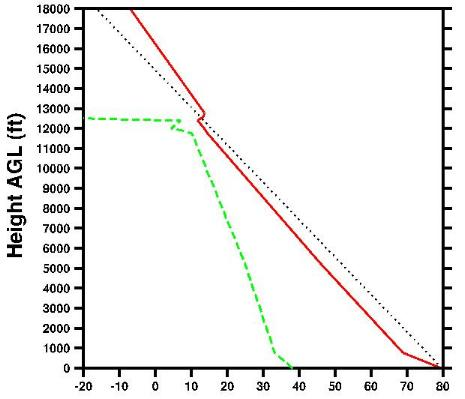The region of air mixed by thermals is called the atmospheric convective Boundary Layer.  The figure gives an example of a convective Boundary Layer (BL), from a 00Z radiosonde observation taken at Reno on a strongly convective day.  The solid red line gives the temperature profile while the dashed green line is the dew point temperature and the dotted black line is a DALR adiabat which begins ar the radiosonde surface temperature.   Features distinctive of strong thermal action are:  (1) the unstable temperature lapse rate immediately above the surface, which is called the surface layer,  (2) the layer of well-mixed air above that, called the mixed layer, which can be divided into a lower region with a lapse rate roughly equal to the DALR up to about mid-BL and an upper region of slightly stable lapse rate, and (3) a very stable layer just above the top of the mixed layer, which marks the top of the thermals and is called the "capping inversion" (though its lapse rate is not always so stable that the temperature actually increases with height).  On weaker thermal days,  (1) the region of unstable lapse rate near the surface will be thinner, and possibly not observable on a sounding,  (2) the temperature difference between the surface temperature and the mixed-layer temperature will be smaller,  (3) the mixed layer will be slightly stable throughout, with no approximately DALR region, and  (4) the capping inversion will be weaker and probably not detectable.  The decrease of dew point temperature with height, such that it approaches the temperature at the mixed-layer top, is common in a convective boundary layer.  Here the dew point is within 4°F of the temperature line near the mixed-layer top, so extensive cloud formation, such as is associated with overdevelopment, is possible at that height since a difference of 4°F (2°C) is typical of cloud formation. (but note that a larger temperature-dewpoint difference can still produce scattered clouds).  Note that the air above the mixed layer is relatively dry and the dew point decreases dramatically above the mixed-layer top - this often occurs, since the surface is a source of both moisture and heat and both are carried upward by the thermals, and can be a distinctive secondary indication of the thermal tops.

The maximum thermalling height is expected to be somewhat lower than the top of the mixed layer.  For this case the TI=0 adiabat based upon the surface temperature coincides, somewhat fortuitously, with the middle of the capping inversion, so the actual mixed-layer top is associated with a TI value just slightly below TI=0.  However, with stronger (weaker) mixing the unstable layer near the surface will be deeper (shallower) and the TI=0 adiabat will then lie to the right (left) of the capping inversion, in which case the TI=0 height will overpredict (underpredict) the thermalling height and a lower (higher) TI value is then associated with the thermalling height.  In general, on more strongly convective days the thermalling height will be associated with a smaller TI value than on weaker convective days.

(I should note that the features described above are more apparent in observed soundings than in the TIP plots since the latter have coarser vertical resolution.  But even observed sounding plots do not depict the exact temperature profile in the mixed layer since not all measured temperatures are plotted, only temperatures at "significant levels".  For example, the atmospheric temperature profile in the above figure should be more rounded than the straight-line segments portray.)

### Development of the Convective Boundary Layer

To understand the TI method, and particularly to interpret a PM plotted sounding, the user needs some understanding of how the temperature structure close to the ground develops with time on a typical day with convective thermals.  In the morning thermals are not present so their mixing influence is not yet felt.  Typically the AM lapse rate near the ground will be relatively stable and unmixed, an example being the early AM sounding shown below - but occasionally strong winds may produce an initial mixed layer.  As the sun rises and surface heating begins, thermals develop and mix the air close to the ground, eroding the stable layer from below to form a mixed layer with approximately a DALR.  As the heating continues the thermals grow in strength and height, mixing more deeply.  The depth of the mixed layer, or approximately DALR region, then increases as the thermals continually erode into the stable atmosphere above the mixing region.  It is important to recognize that mixed-layer top, i.e. the top of the approximately DALR region, coincides with the thermal tops (since the thermal is creating the mixing) and this is (roughly) the expected maximum thermalling height.  Generally the lapse rate in the mixed layer is closest to the DALR sometime after noon, when the mixing is strongest; at other times the lapse rate is slightly more stable.  In the afternoon the thermals weaken somewhat but because a neutral stability mixed layer is already present, and thus resistance to vertical motion is weak, they still tend to maintain the existing mixed-layer depth.  Near sunset, with a loss of surface heating, the thermals die out and the temperature structure becomes increasingly more stable.

## Note:  For simplicity the plots below use Temperature as the horizontal axis and Height as the vertical axis.  The same principles can be utilized in analyzing a "SkewT" diagram in which the Temperature axis is skewed from the horizontal and Pressure is the vertical axis - but the SkewT plots will look, er, skewed from those presented below.

### the Thermal Index method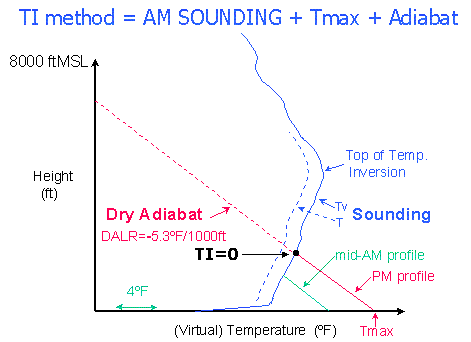The TI (Thermal Index) method idealizes the convective boundary layer to estimate the mixed-layer top, and thereby the maximum thermalling height.  For an initial morning sounding and a predicted maximum surface temperature (Tmax), the TI method simply assumes that the afternoon temperature profile will be a perfectly-mixed DALR layer.  A DALR adiabat line is therefore extended from the surface Tmax until it intersects the AM sounding profile, to give the level where TI=0.  Air below that height is considered to be well mixed by the thermals, while air above that height is considered unaffected.  Thus the TI method "predicts" how a PM temperature profile will differ from the AM temperature profile, but it assumes that the difference results only from thermal mixing and that the mixing will be perfect.  The maximum thermalling height is then assumed to be at or somewhat below the TI=0 height.

The numerical Thermal Index at any height is the difference between the AM sounding temperature at that height and the "predicted" PM temperature at that height (so negative TI values are found below the TI=0 height).  Every height has a TI "trigger temperature": when the surface temperature reaches that trigger temperature the TI method predicts that mixing will then reach that height, i.e. then TI=0 at that height.

The Tmax in above example is at an elevation equal to the sounding's release elevation, i.e. the surface is assumed to be flat.  The TI method can approximate the mixing over nearby elevated terrain by starting the DALR adiabat at that terrain's elevation with its expected Tmax.  This assumes that the valley sounding is also applicable to the elevated terrain, which in many cases is a reasonable approximation.

The TI method can also be used with model PM soundings to estimate thermalling heights.  However, the TI method assumes that the sounding temperature profile represents an unmixed atmosphere, so numerical TI values from PM model soundings are meaningful, and hence valid, only for heights above the top of the mixed layer predicted by the model.  (Being a valid prediction is not the same as saying that it is a correct prediction - the latter depends upon whether the TI-assumed Tmax is accurate!)  This also implies that the TI method is generally invalid and should not be used if the Tmax utilized for the TI adiabat is smaller than the model-predicted Tmax, since in such a case the adiabat from the TI-assumed Tmax will not intersect the sounding temperature profile below the top of the mixed layer predicted by the model (and may not intersect the temperature profile anywhere!).

### model predicted PM soundings

While the TI method uses idealized mixing assumptions to predict PM temperature profiles, numerical weather models predict PM temperature profiles by using complex differential equations which include many factors besides mixing.  Starting from an AM "initialization" profile, the model marches forward in time, calculating the temperature change at specific levels corresponding to model grid points.  The model calculations tend to produce a "well mixed" profile when thermal mixing occurs, but just as mixing is seldom perfect in the real atmosphere the "well mixed" profiles predicted by the models are often not perfectly mixed.  In addition, the PM model predictions include changes occurring above the mixed layer due to effects such as downward atmospheric motion (subsidence), effects which affect afternoon soaring but which are omitted when one considers only the AM sounding.

Analysis of a PM model sounding plot is the best method of estimating the maximum thermalling height for that soaring day.  Due to the variety of thermal profiles that can occur, using the brain's pattern recognition capability to visually determine the predicted top of the mixed layer will provide a better estimate than any summary number that a computer can calculate.  Additionally, the human eye can judge the degree to which the atmosphere is predicted to be truly "well mixed": if the predicted lapse rate is noticeably less than the DALR then the thermalling can be expected to be degraded.   Cloud bases can also be estimated for overcast or near overcast conditions (scattered clouds are difficult to predict visually from a sounding, but are more likely as the difference between the predicted temperature and dew point temperature decreases).  However atmospheric complexity and model limitations can produce interpretation difficulties.  For example,  estimating the top of the mixed layer can be ambiguous when the lapse rate above the mixed layer is not very different from the lapse rate in the mixed layer.  Still, a computer summary estimate is always more subject to error than is a knowledgeable eyeball estimate.  One caveat is that PM model soundings used in the TIP represent conditions at 00Z, after the time of maximum heating, and thus their surface temperature is below the day's Tmax and their maximum thermal height tends to be somewhat underpredicted.  Also, of course, they are only model predictions - the real atmosphere may differ!

### Clouds

I need to say something about clouds, since their effects will appear in sounding profiles, but I will say as little as possible.  Clouds form when cooling reduces the atmospheric temperature to the dew point temperature, condensing water vapor into liquid water.  This condensation releases heat aloft, increasing the buoyancy of the thermals and altering the lapse rate of a perfectly mixed cloud to become the MALR [Moist Adiabatic Lapse Rate] instead of the DALR.  The MALR is always larger than the DALR, which would be interpreted as being "stable" if the atmosphere were dry (but it is not).

Condensation is considered likely when the difference between the atmospheric temperature and the dew point temperature is about 4°F (2°C).  Remembering that model predictions are for area-averages, "extensive clouds" (overcast or broken clouds) will be indicated in a sounding when the dew-point temperature is within 4°F of the atmospheric temperature and the temperature slope will then be the MALR), i.e. slightly more "stable" than the DALR.  Scattered cloud conditions cannot be adequately predicted from the TIP sounding plot, since it lacks lines of constant humidity mixing ratio.  [As an aside, if a sounding is plotted on a SkewT diagram then the absence/existence of BL clouds can be crudely predicted by noting whether the dew-point temperature of the maximum humidity mixing ratio within the BL, which typically occurs at the surface, intersects the atmospheric temperature anywhere within the BL: if it does not then no clouds are predicted, whereas if it does then clouds formation is predicted with the degree of sky coverage increasing as the overlap increases.].  I should note that moisture (and hence cloud) prediction is a notable weakness of meteorological models, so cloud predictions should be taken with a large grain of salt.

### TI vs Model PM height prediction

The TIP PM sounding plots actually give two predictions for the PM temperature profile: one from the TI method, using a NWS predicted surface temperature with an idealized mixed-layer profile, and the other predicted by a model, using its internally computed surface temperature and temperature profile.  Which is the "best" estimate?  Life, or at least meteorology, is not simple and the answer is "neither".  I have more faith in the NWS predicted Tmax than in the model-predicted surface temperature Tsfc, because the former is often "tuned" to a particular location whereas the model temperature is more generic.  However, the shape of the model temperature profile is more likely to agree with the actual atmospheric profile than is the idealized straight line assumed by the TI method.  Therefore, I try to meld the two profiles together, mentally shifting the model prediction such that at the lowest plotted elevation its temperature matches the TI method temperature, and thereby estimate the resulting top of the mixed layer, which I assume to be the maximum thermalling height.

When the TI-predicted Tmax is warmer than the model-predicted Tsfc,  this generally means that the expected mixed-layer top, and thus the expected maximum thermalling height, is just below the TI=0 height.  When the TI-predicted Tmax is cooler than the model-predicted Tsfc, then the model-predicted mixed-layer top must be decreased accordingly to give the expected mixing height.  Remeber that numerical TI values from PM model soundings are valid only for heights above the top of the mixed layer predicted by the model, so the TI method should not be used if the actual surface temperature is believed to be smaller than the model-predicted surface temperature.

### Example Interpretations

I will evaluate several example PM MAPS model soundings as plotted in the TIP format to illustrate how such plots can be analyzed.  In these plots height is the vertical axis and temperature is the horizontal axis.  No numerical scale is given for the temperature axis, but each column represents 1°F.  Circles represent the predicted model (virtual) temperature at 500 ft height increments.  Dots depict the predicted dew point temperature.  The plots also include the TI-method estimate of the PM temperature profile plotted as asterisks, i.e. a DALR adiabat extending from the surface Tmax predicted by the NWS (not by the model). The slope of the adiabat indicates the (approximate) slope expected for any mixed layer in the sounding.  And finally, note that the limited resolution of the plots and of the models means that details of the mixed layer are often smoothed out; observed soundings have higher resolution and the features described in the "Convective Boundary Layer" section above are more apparent in those soundings than in the present plots (but even observed sounding plots do not depict the exact temperature profile in the mixed layer since they do not plot all measured temperatures but only temperatures at "significant levels")  Note that the model predicts its own surface temperature, which I will call Tsfc, which may differ from the Tmax assumed for the TI-method adiabat.  Also note that the plots only extend down to 500 ft and therefore omit the surface itself.  And finally, note that the limited resolution of the plots and of the models means that details of the mixed layer are often smoothed out so predictions of the maximum thermalling height have a "fuzziness" of around 500 ft.

Avenal (Lemoore), Jan 30, 2001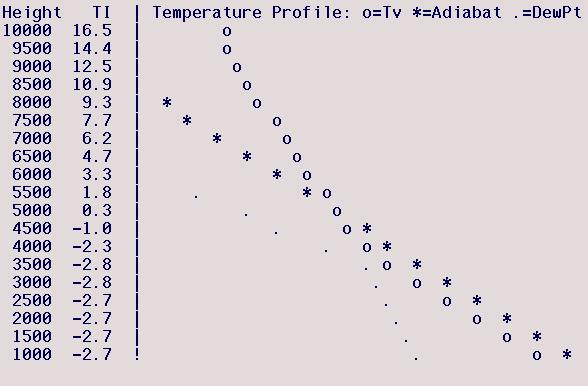This case is similar to the strongly convective boundary layer illustrated above. The model lapse rate is well-mixed, essentially a DALR, up to 3500 ft.  The model-predicted top of the mixed layer is best determined by noting that the region above the DALR region has an essentially constant lapse rate that intersects with the DALR region around 4000 ft.  The dew point drops dramatically above 3500 ft, suggesting a mixing height between 3500-4000 ft.  So the best estimate of the model-predicted maximum thermalling height is between 3500-4000 ft.  The dew point is very close to the atmospheric temperature at 3500 ft, indicating that thin clouds may form at the top of the mixed layer.  The model-predicted Tsfc is slightly lower than the TI-assumed Tmax (a NWS prediction), by about 3°F (3 columns).  The warmer TI-assumed Tmax predicts a somewhat higher mixed-layer top of  4750 ft, which is the TI=0 height - note this is above the model-predicted mixed-layer top and is therefore a meaningful TI prediction for the TI-assumed Tmax .  Melding the two predictions together, and relying on the TI-method's NWS Tmax to be a more accurate surface temperature prediction, I would estimate the maximum thermalling height to be around 4250 ft.

Avenal (Lemoore), Mar 28, 2001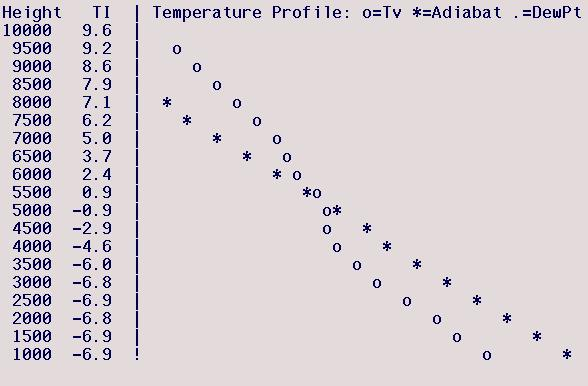The model predicts a nearly DALR (actually very slightly stable) mixed layer extending to a height of around  3000 ft, above which the stability increases somewhat before becoming very stable between 4500 and 5000 ft.  The latter marks the capping inversion, so the top of the model's mixed layer is around 4500 ft.  Note that the model's Tsfc is significantly lower than the TI-assumed Tmax, which predicts a mixed-layer top (TI=0) of 5250 ft (meaningful because it is above the model-predicted mixed-layer top).   Melding the two predictions together, and placing more weight on the TI-assumed Tmax, I would estimate a maximum soaring height of around 5000 ft.

Williams (Marysville), Mar 27, 2001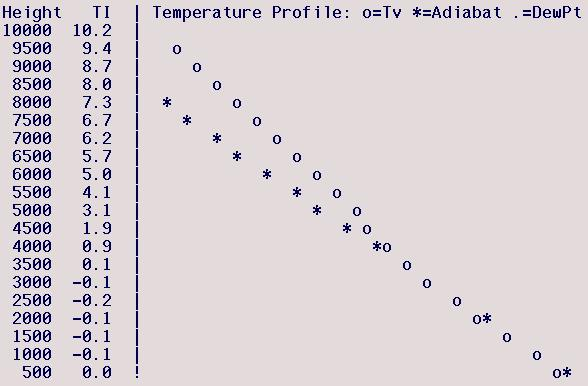The PM model sounding has a nearly DALR mixed layer up to a height of 4000 ft.  The lapse rate becomes slightly stable up to 4500 ft, then a more strongly stable region exists between 4500-5000 ft, above which there is a deep layer of  slight stability.  Determining the top of the model's mixed-layer In cases such as this where the lapse rate above the mixed layer does not differ markedly from the lapse rate in the upper mixed layer can be difficult, but here the more stable layer seems to be a capping inversion so I estimate the top of the model-predicted mixed layer to be 4500 ft.  Here the model-predicted Tsfc and TI-assumed Tmax are very close.  There are actually two TI=0 heights, at 900 and 3500 ft (see the TI column since the circles overplot the asterisk) but neither are meaningful since they are below the model-predicted mixed-layer top, and thus are not meaningful predictions.  So the estimated thermalling height is the model's mixed-layer top, 4500 ft.

Avenal (Lemoore), Feb 10, 2001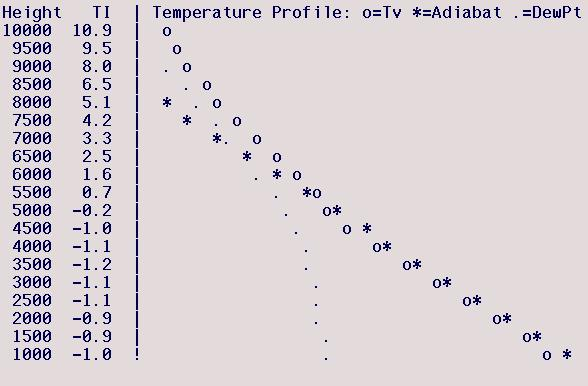Just above the surface the model-predicted lapse rate is nearly DALR up to 4500 ft, above which it is moderately "stable".  However, 5000 ft appears to be a cloud base since the dew point there nears the atmospheric temperature and nearly parallels it above that - so  thermals likely extend above 5000 ft and the "stable" lapse rate there is really the adiabatic lapse rate (MALR).  Here the maximum soaring height will be below 5000 ft due to the cloud base.

Hollister (Oakland), Mar 22, 2001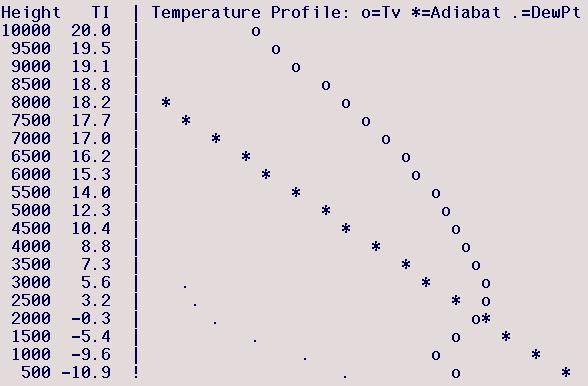The model predicts a shallow mixed layer up to 1000 ft, above which is a strongly stable inversion layer.  The TI-assumed Tmax is much larger than the model-predicted Tsfc.  This forecast is for Hollister so I greatly favor the NWS Tmax predictions, since the model Tsfc predictions are for an Oakland location, and would predict the top of the mixed layer to occur at the TI=0 height between 2000-2500 ft.  Note that TI =0 height is above the model-predicted mixed-layer top and is thus meaningful.

Avenal (Lemoore), Feb 6, 2001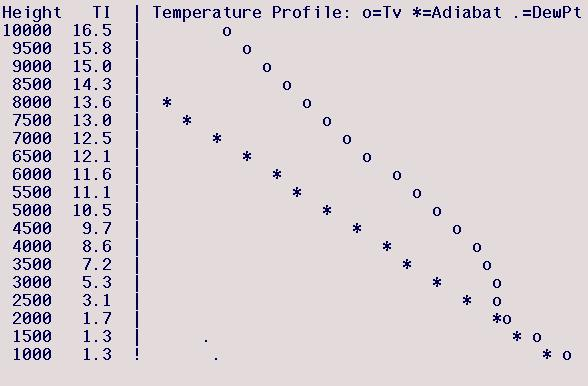The model-predicted well-mixed DALR layer extends to 2000 ft, above which the sounding is strongly stable, so the model's mixed-layer top is between 2000-2500 ft.  Note that the TI adiabat never intersects the model-predicted temperature profile so there is officially no TI=0 height - this occurs because the TI-assumed surface temperature is cooler than the model-predicted surface temperature in which case use of the TI method is generally invalid, as occurs here.  One's eyeball indicates that the model-predicted temperature profile is just slightly warmer than the the TI-predicted adiabat so the model-predicted mixed-layer temperature is essentially correct and its mixed-layer top is also essentially correct, though slightly greater than would be predicted if the model Tmax equalled the NWS predicted Tmax.  Weighting the NWS Tmax more heavily here means that the expected thermalling height will be slightly below the model-predicted mixed-layer top, and I would estimate it to be 2000 ft.

Williams (Marysville), Jan 31, 2001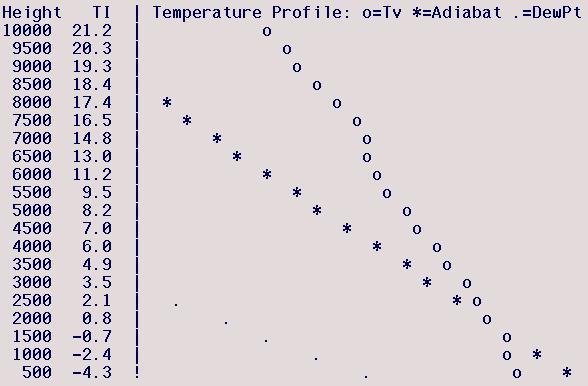Here the model-predicted profile has no well-mixed layer.  The model's lapse rate between 500-1000 ft is moderately stable, with a very stable region between 1000-1500 ft.  The latter may or may not be a capping inversion, but would indicate a mixed-layer top of 1000 ft.  In any event, since the layer below that is not well-mixed any thermals are predicted to be weak.  The TI-assumed Tmax is significantly larger than the model-predicted Tsfc and predicts a TI=0 mixed-layer top of 1750 ft.  This is a meaningful prediction because this height is above the model-predicted mixed-layer top, so I would meld the two profiles together, weighting the TI-assumed Tmax more heavily, to predict a maximum thermallling height of 1500 ft.  But the model's prediction of very weak thermals indicates the thermalling will be even worse than the low mixed-layer top would indicate.  This is definitely not a day to expect to do any thermalling!